# Mixture models: motivation

• new modellers have an incorrect mental model for representative situations where mixture models are used
• we are going to look at a simplified version of a real world Bayesian model today
• in which mixtures play a key role
• joint work with collaborators at BC Cancer (Sam Aparicio, Peter Eirew, Andrew Roth)

# Mixture models: motivation

Setup molecular barcodes in cancer cells

• patient derived breast cancer samples
• CRISPR-Cas9 is used to:
• edit genome of cancer cell (about 200 genes targeted, we look at only 1 of them)
• many cells get the same edit, but we also embed a unique molecular identifier in individual cells
• sample is then implanted in immuodeficient mouse
• after a few week, tumour has grown and is sequenced

Note when the cell divide, the barcode gets copied. So at the end of the process, each “unique” barcode is no longer unique! At the end of the process, the number of times we sequence each barcode gives us an indication of the number of progenies the original CRISPR edited cell gave rise too. Hence a notion of “family size”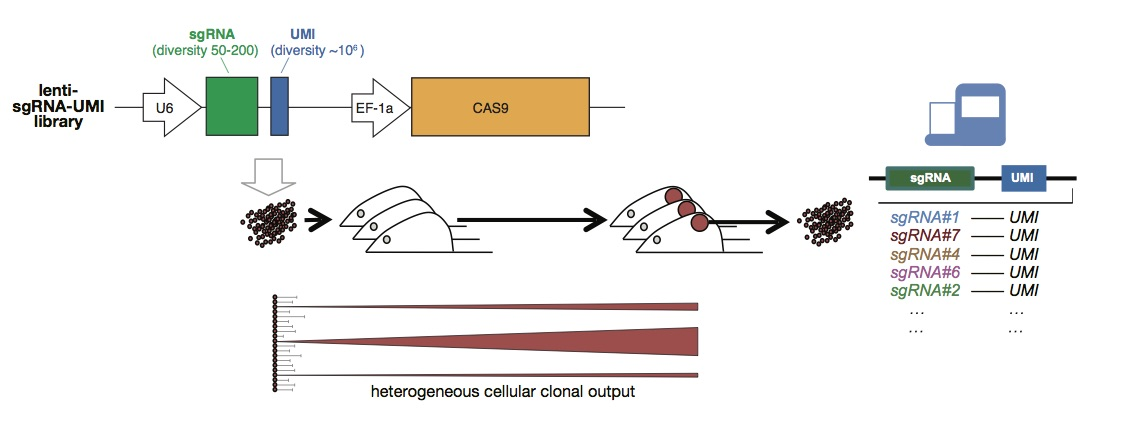Data the number of times we sequenced each barcode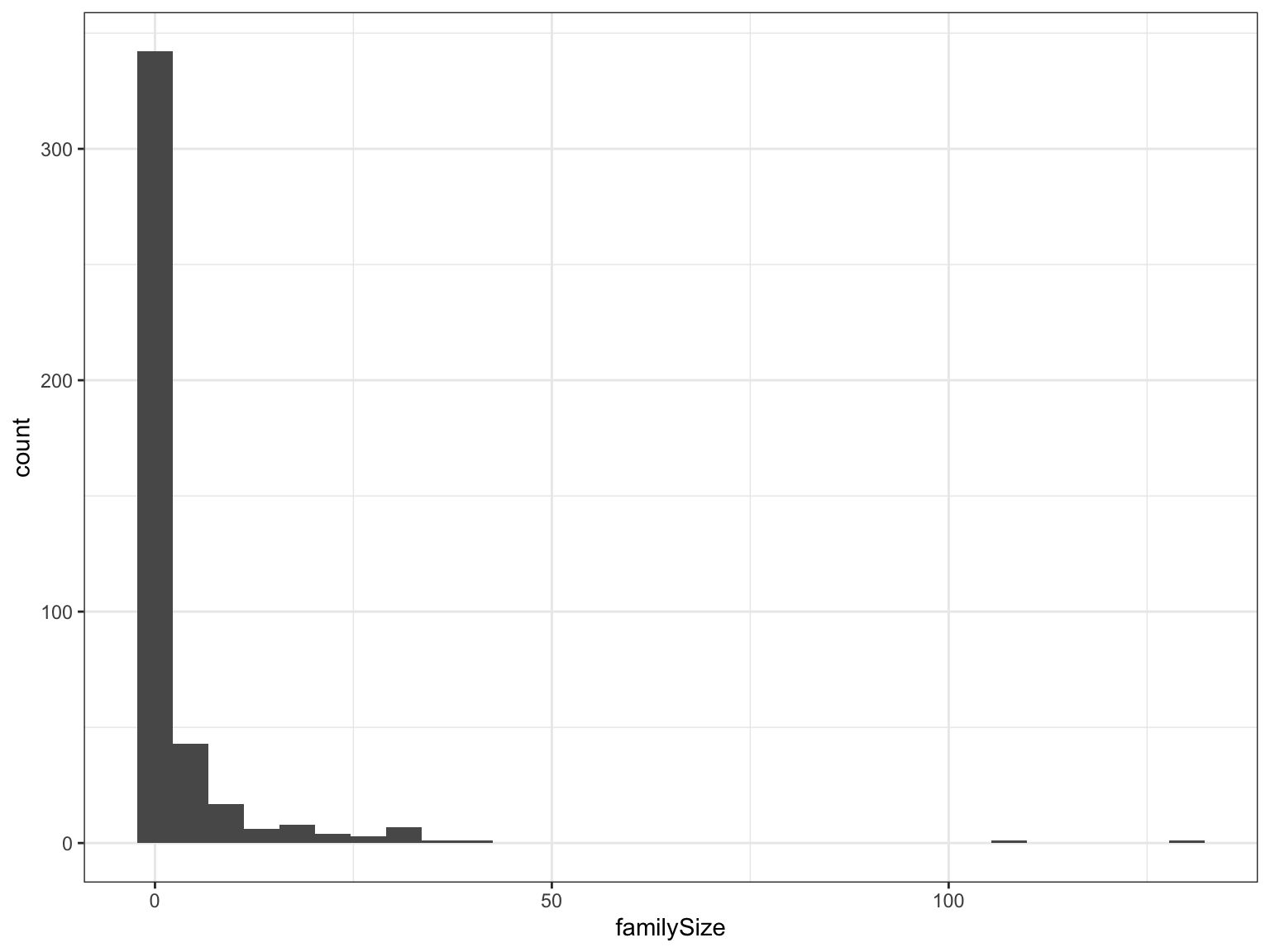Challenges

• we do not have a theory (yet) that provides a distribution family for the likelihood of the data
• as we will see, default choices, for example geometric, Poisson, or even negative binomial, do not work well (hint of the root cause: presence of extremes)

### Poll: How to check if say a Poisson is a good choice?

1. Posterior predictive checks
2. Compute Bayes factors
3. Leave one out probability integral transform
4. Simulation based calibration
5. None of the above

# Goodness-of-fit checks!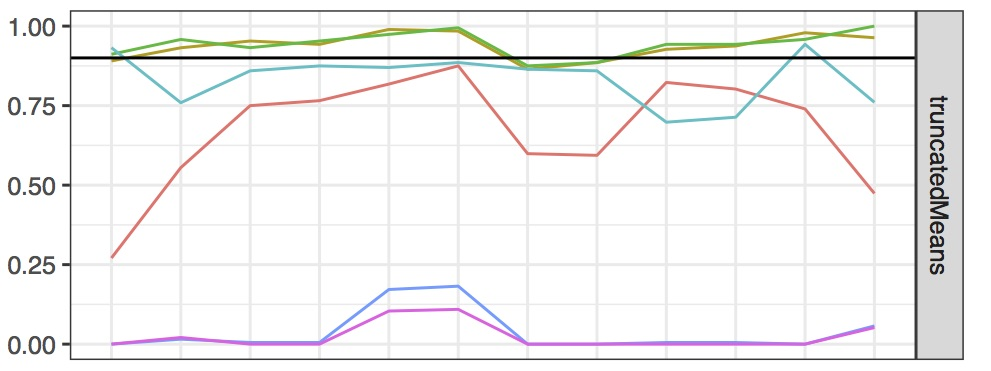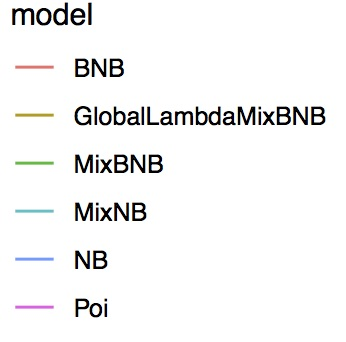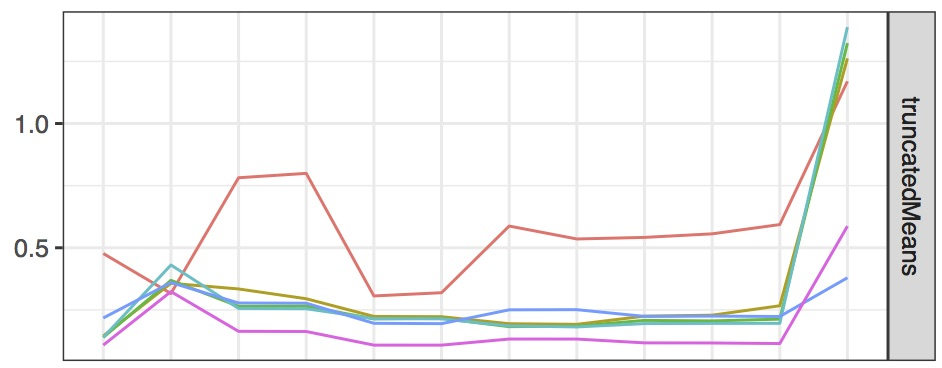• Each dataset has several approximately iid copies (one for each targeted genes)
• Used a simple posterior predictive check (no leave-one-out used here)
• Here we looked at an observed statistic “truncatedMean”
• Built credible interval for the observed statistic
• Checked fraction of times observed value is in the interval
• Plot above:
• Black line: nominal (target) coverage
• Report, for different models and datasets, the actual coverage
• Note: the standard choices in bioinformatics, Poisson (Poi, 1 param) and Negative Binomial (NB, 2 param), catastrophically fail!
• We first looked at slightly more expressive distribution that can produce fatter tails: e.g. the Beta Negative Binomial distribution (3 params), which for some parameter combinations gives infinite means
• But that still fits the data very poorly!
• At this point we are running out of “named distributions”

# Mixture

• Solution: use mixtures of known distributions
• Example: MixNB, a mixture of two Negative Binomial distributions, each with a cluster-specific parameter vector $$\theta_i = (\theta_{i,1}, \theta_{i,2})$$

$f_{\text{MixNB}}(y;\theta_1, \theta_2, p) = p f_{\text{NB}}(y; \theta_1) + (1-p) f_{\text{NB}}(y; \theta_2)$• Examples of priors on $$p$$: uniform or beta.

# Finite mixtures

• This is an example where the number of “clusters” $$K$$ is set to two.
• Generalization to finite $$K > 2$$:
• $$p$$ is replaced by $$(p_1, p_2, \dots, p_K)$$, $$\sum p_i = 1$$ (mixture proportions)
• Typical prior on $$p$$: Dirichlet distribution (not to be confused with Dirichlet process!)
• How to pick $$K$$?
• First route: use model selection techniques

# Infinite mixtures

• Second route: “infinite mixture of finite mixtures” (MFM), where we make $$K$$ random, for example
• $$K \sim \text{Poi}(1)$$ (or any other integer distribution)
• $$p | K \sim \text{Dirichlet}(1_K )$$ where $$1_K$$ a one-vector of length $$K$$
• Third route: “Bayesian non-parametric methods” (BNP) set $$K = \infty$$, and use a distribution on $$p$$ that encourages datapoints to share parameters

# MFM versus BNP

• Let $$P_n$$ denote the number of parameters used to generate $$n$$ datapoints
• by generate, we mean “forward simulation a dataset”, see Graphical model lecture
• Note: this is distinct from the number of clusters $$K$$, since some clusters might not get used up
• Let $$P = \lim_{n\to \infty} P_n$$
• Note: $$P_n$$ are $$P$$ are random!

• Distinction
• Bayesian parametric model and mixture of finite mixtures: $${\mathbb{P}}(P = \infty) = 0$$
• Bayesian non-parametric model (BNP): $${\mathbb{P}}(P = \infty) > 0$$.

# Mixture models: a second application

• So far, we have focussed on using mixture models to create more expressive models
• For example, the CRISPR example where Poisson, NB, and any named distribution was grossly misspecified
• In simple setups, this type of application is called “density estimation”
• There is another use of mixture models: “clustering”
• To understand that second use, we need to re-express our mixture graphical model into another equivalent one

# Cluster indicators

Consider these two models (for simplicity, consider $$\theta = (\theta_1, \theta_2), p$$ as fixed for now)

• The mixture we are familiar with
• $$y | \theta, p \sim p f(y; \theta_1) + (1-p) f(y; \theta_2)$$
• The two step process based on a cluster indicators $$z$$
• $$z | p \sim {\text{Bern}}(p)$$
• $$y | z, \theta \sim f(\cdot; \theta_z)$$

For concretess, just as an example let us say $$f$$ is Poisson, $$\theta = (1, 2)$$, $$p = 1/2$$. Let $${\mathbb{P}}_\text{mix}$$ and $${\mathbb{P}}_\text{clust}$$ denote these two models

### Poll: compare $${\mathbb{P}}_\text{mix}(Y = 7)$$ and $${\mathbb{P}}_\text{clust}(Y = 7)$$

1. In both cases $$Y$$ is marginally Poisson-distributed, so the two probability are equal
2. The two probability are equal for other reasons
3. $${\mathbb{P}}_\text{mix}(Y = 7) < {\mathbb{P}}_\text{clust}(Y = 7)$$
4. $${\mathbb{P}}_\text{mix}(Y = 7) > {\mathbb{P}}_\text{clust}(Y = 7)$$

# Cluster indicators

• The two models probabilities are always equal (not just for $$Y=7$$)
• The fact that the random variables are Poisson has nothing to do with it (do not confuse $$Y = Y_1 + Y_2, Y_i \sim \text{Poi}(\theta_i)$$ versus a mixture, $$Y = Y_1 {{\bf 1}}[Z = 0] + Y_2 {{\bf 1}}[Z = 1]$$)

\begin{align*} {\mathbb{P}}_\text{mix}(Y = 7) &= \sum_{z=0}^1 {\mathbb{P}}_\text{mix}(Z = z) {\mathbb{P}}_\text{mix}(Y = 7 | Z = z) \\ &= p f(y; \theta_1) + (1-p) f(y; \theta_2) \\ &= {\mathbb{P}}_\text{clust}(Y = 7) \end{align*}

Terminology we have two representations for the same model

# Model based clustering

• Motivation: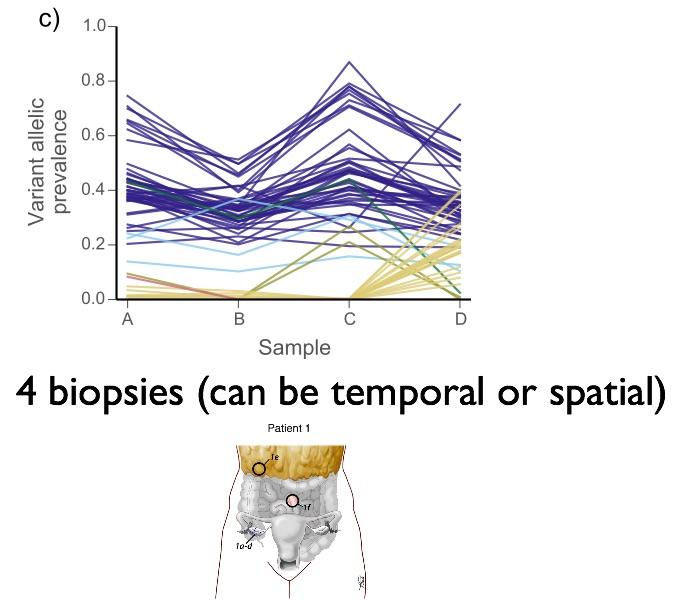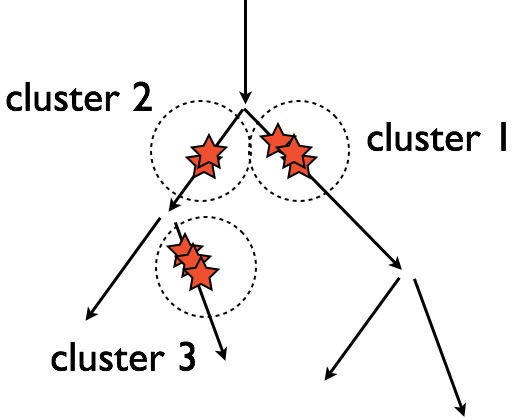• Idea: use the cluster indicators (one associated to each data point), to form a grouping/clustering/partition of the observations
• $$p \sim \text{Beta}$$
• $$\theta_i \sim \dots$$
• For each observation $$i$$:
• $$z_i | p \sim {\text{Bern}}(p)$$
• $$y_i | z_i, \theta \sim f(\cdot; \theta_{z_i})$$

# Summarization of the posterior and label switching

### Summarization

• From MCMC, we obtain a collection of cluster indicator samples $$z^{(1)}, z^{(2)}, \dots, z^{(10000)}$$, each is a vector with same length as dataset: $$z^{(m)} = (z^{(m)}_1, \dots, z^{(m)}_n)$$
• How to summarize into one clustering $$z^*$$?
• Pitfall: label switching

### Label switching

• Often, in mixture models, both the prior and likelihood are exchangeable in terms of the cluster index $$k$$ $$\Longrightarrow$$ the posterior is exchangeable in $$k$$
• I.e. relabelling all instance of clustering $$k=0$$ to $$k=1$$ and vice versa
• Problem can be even more serious when we have “approximate exchangeability” instead of exact exchangeability
• However, that exchangeability might not be detected in short MCMC runs; more advanced methods such as parallel tempering and sequential change of measure may be needed to detect them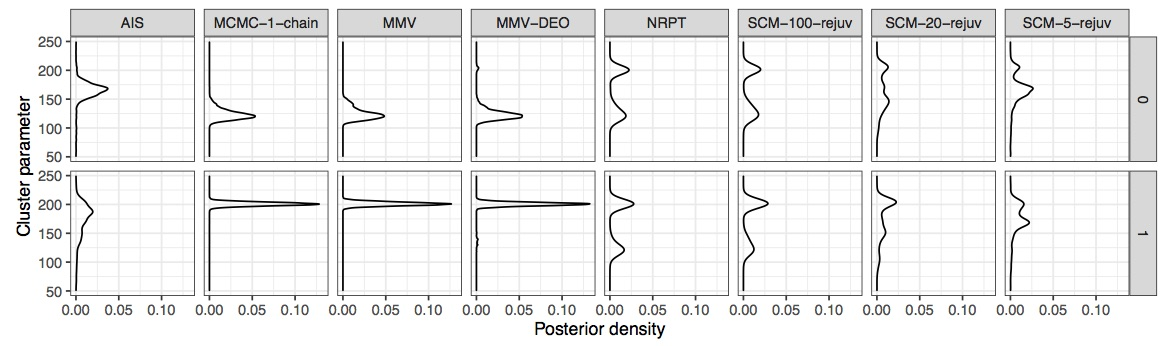• Pitfall: using summarization method based on a function $$f(z)$$ where $$f$$ is sensitive to cluster relabelling

### Poll: how to obtain a summarization method that is invariant to cluster relabelling

1. Use the mean of each $$z_i$$
2. Use the median of each $$z_i$$
3. Use the mode of each $$z_i$$
4. Something else

# Example of a summarization method that is invariant to cluster relabelling

• Use the Bayes estimator!
• To define a loss, instead of thinking of the clustering as a vector of integers, $$z$$, view it as a partition (set of disjoint sets) of the $$n$$ datapoints
• True partitions $${\rho}$$
• Guessed partition $${{\rho'}}$$
• rand loss function is denotes as $${\textrm{rand}}({\rho}, {{\rho'}})$$.

$\sum_{1\le i < j \le n} {{\bf 1}}[(i \sim_{\rho}j) \neq (i \sim_{{\rho'}}j)],$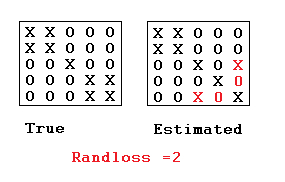Goal: computing the Bayes estimator derived from the rand loss.

First, we can write:

\begin{aligned} {\textrm{argmin}}_{\textrm{partition }\rho'} {\mathbf{E}}\left[{\textrm{rand}}(\rho, \rho')|X\right] &= {\textrm{argmin}}_{\textrm{partition }\rho'} \sum_{i<j} {\mathbf{E}}\left[{{\bf 1}}\left[\rho_{ij} \neq \rho'_{ij}\right]|X\right] \\ &= {\textrm{argmin}}_{\textrm{partition }\rho'} \sum_{i<j} \left\{(1-\rho'_{ij}){\mathbb{P}}(\rho_{ij} = 1|X) + \rho'_{ij} \left(1- {\mathbb{P}}(\rho_{ij} = 1 |X)\right)\right\} \end{aligned}

where $$\rho_{i,j} = (i \sim_{\rho} j)$$, which can be viewed as edge indicators on a graph.

The above identity comes from the fact that $$\rho_{i,j}$$ is either one or zero, so:

• the first term in the the brackets of the above equation corresponds to the edges not in the partition $$\rho$$ (for which we are penalized if the posterior probability of the edge is large), and
• the second term in the same brackets corresponds to the edges in the partition $$\rho$$ (for which we are penalized if the posterior probability of the edge is small).

This means that computing an optimal bipartition of the data into two clusters can be done in two steps:

1. Simulating a Markov chain, and use the samples to estimate $${s}_{i,j} = {\mathbb{P}}(\rho_{ij} = 1 | Y)$$ via Monte Carlo averages.
2. Minimize the linear objective function $$\sum_{i<j} \left\{(1-\rho_{ij}){s}_{i,j} + \rho_{ij} \left(1- {s}_{i,j}\right)\right\}$$ over bipartitions $$\rho$$.

Note that the second step can be efficiently computed using min-flow/max-cut algorithms (understanding how this algorithm works is outside of the scope of this lecture, but if you are curious, see CLRS, chapter 26).

# Another pitfall

• We have seen two applications of mixture models:
• Density estimation (we don’t care about $$z$$, we just want a more flexible likelihood)
• Clustering (we care about $$z$$)
• We have seen two classes of infinite mixtures, depending on $$P_n$$, the behaviour of the number of parameters used to $$n$$ datapoints, and its limit $$P = \lim_{n\to \infty} P_n$$
• Mixture of finite mixtures (MFM) $${\mathbb{P}}(P = \infty) = 0$$
• Bayesian non-parametric model (BNP): $${\mathbb{P}}(P = \infty) > 0$$
• For many BNP models, in fact, $${\mathbb{P}}(P = \infty) = 1$$.
• So this means that if there is a true finite number of clusters, i.e. $$K < \infty$$ and $$P < \infty$$, we are putting zero prior mass on the event $$(P < \infty)$$
• Hence if you are using BNP for clustering, you can easily get a procedure trying to estimate $$K$$ which is not consistent
• See: https://arxiv.org/abs/1301.2708
• So the pitfall is to use e.g. DP for finding $$K$$
• Literally thousands of papers fall in that pitfall
• Note that BNP still valuable for the density estimation application where they excel